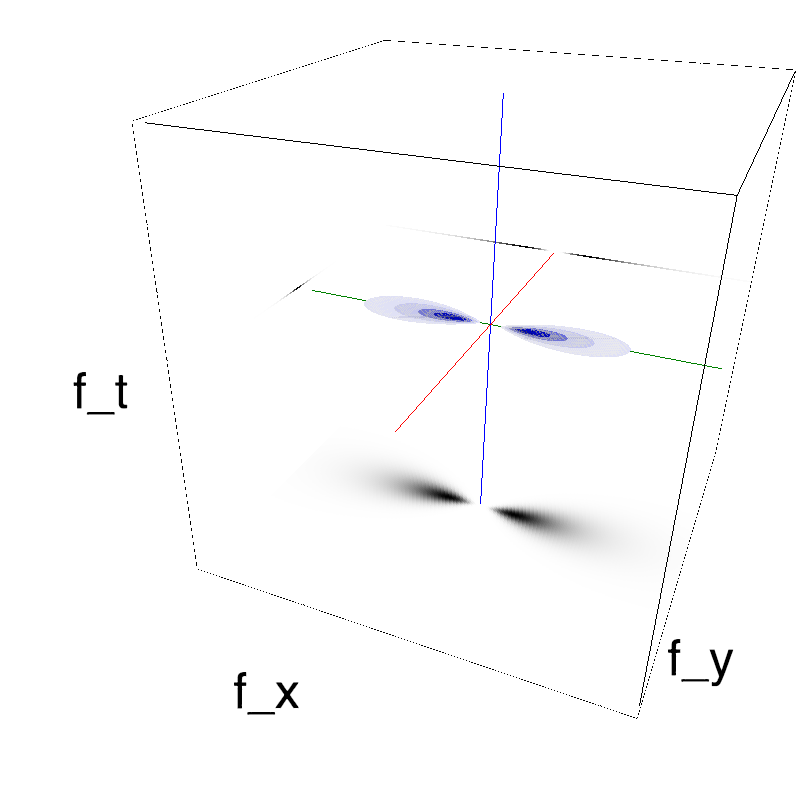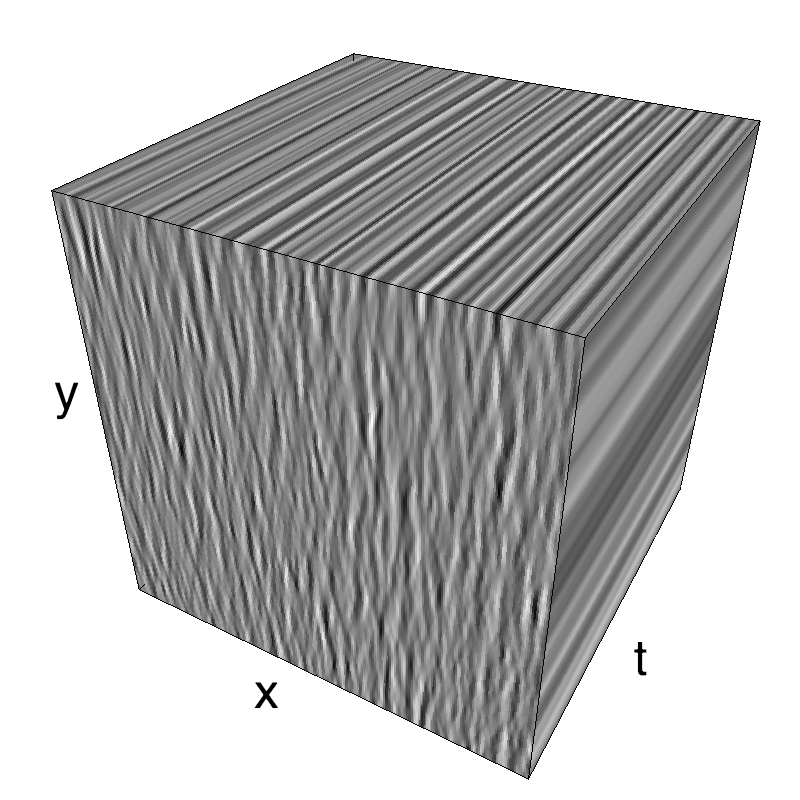# Static Motion Clouds

Motion Clouds were originally defined as moving textures controlled by a few parameters. The library is also capable to generate a static spatial texture. Herein I describe a solution to generate a single static frame.

There are multiple solutions, and the simplest is perhaps to generate a movie that does not move. This means that the mean velocity $(V_X, V_Y)$ is null $=(0, 0)$ but also that there is no noise in the definition of the speed plane. This is defined by $B_V=0$, that is, that all the energy is concentrated on the spped plane:

In :
import MotionClouds as mc
fx, fy, ft = mc.get_grids(mc.N_X, mc.N_Y, mc.N_frame)
name = 'static'
env = mc.envelope_gabor(fx, fy, ft, V_X=0., V_Y=0., B_V=0)
mc.figures(env, name)
mc.in_show_video(name)

/usr/local/lib/python3.7/site-packages/vispy/visuals/isocurve.py:22: UserWarning: VisPy is not yet compatible with matplotlib 2.2+
warnings.warn("VisPy is not yet compatible with matplotlib 2.2+")As such, all frames are the same and it suffices to take one of them.

Note that as a matter of fact there is a "mathematical issue" here: if we define $B_V = 0$ in our implementation we need to take care of the fact that the formula for the Gaussian doest not give a "dividion by zero" error. The MotionClouds code takes this possibility into accountin the mc.envelope_speed function:

In :
help(mc.envelope_speed)

Help on function envelope_speed in module MotionClouds:

envelope_speed(fx, fy, ft, V_X=1.0, V_Y=0.0, B_V=0.5)
Returns the speed envelope:
selects the plane corresponding to the speed (V_X, V_Y) with some bandwidth B_V.

* (V_X, V_Y) = (0,1) is downward and  (V_X, V_Y) = (1, 0) is rightward in the movie.
* A speed of V_X=1 corresponds to an average displacement of 1/N_X per frame.
To achieve one spatial period in one temporal period, you should scale by
V_scale = N_X/float(N_frame)
If N_X=N_Y=N_frame and V=1, then it is one spatial period in one temporal
period. It can be seen along the diagonal in the fx-ft face of the MC cube.

A special case is used when B_V=0, where the fx-ft plane is used as
the speed plane: in that case it is desirable to set (V_X, V_Y) to (0, 0)
to avoid aliasing problems.

Run the 'test_speed' notebook to explore the speed parameters, see
https://neuralensemble.github.io/MotionClouds/posts/testing-speed.html



However, this solution is not the most elegant and one could also just state that N_frame is 1 (that is, that the movie consists of only one frame).

The library is made such that this will also work in this slightly degenerate mode:

In :
mc.N_frame=1
fx, fy, ft = mc.get_grids(mc.N_X, mc.N_Y, mc.N_frame)
name = 'static'
env = mc.envelope_gabor(fx, fy, ft, V_X=0., V_Y=0., B_V=0)
z = mc.rectif(mc.random_cloud(env))
print(z.shape)
z = z.reshape((mc.N_X, mc.N_Y))

(256, 256, 1)


Note that in that case, you need to reshape your matrix to one dimension. Using this numpy array, you can now simply plot it:

In :
import matplotlib.pyplot as plt
%matplotlib inline
fig, ax = plt.subplots(figsize=(10,10))
_ = ax.imshow(z.T, cmap=plt.gray())### a simple application: defining a set of stimuli with different orientation bandwidths¶

In :
import numpy as np
import MotionClouds as mc
import matplotlib.pyplot as plt
downscale = 1
fx, fy, ft = mc.get_grids(mc.N_X/downscale, mc.N_Y/downscale, 1)

N_theta = 6
bw_values = np.pi*np.logspace(-2, -5, N_theta, base=2)
fig_width = 21

fig, axs = plt.subplots(1, N_theta, figsize=(fig_width, fig_width/N_theta))
for i_ax, B_theta in enumerate(bw_values):
mc_i = mc.envelope_gabor(fx, fy, ft, V_X=0., V_Y=0., B_V=0, theta=np.pi/2, B_theta=B_theta)
im = mc.random_cloud(mc_i)

axs[i_ax].imshow(im[:, :, 0], cmap=plt.gray())
axs[i_ax].text(5, 29, r'$B_\theta=%.1f$°' % (B_theta*180/np.pi), color='white', fontsize=32)
axs[i_ax].set_xticks([])
axs[i_ax].set_yticks([])
plt.tight_layout()
fig.subplots_adjust(hspace = .0, wspace = .0, left=0.0, bottom=0., right=1., top=1.)

#import os
#fig.savefig(os.path.join('../figs', 'orientation_tuning.png'))